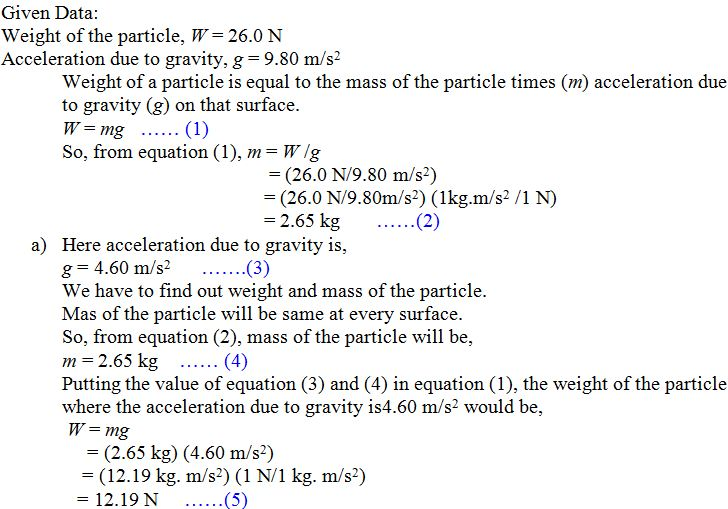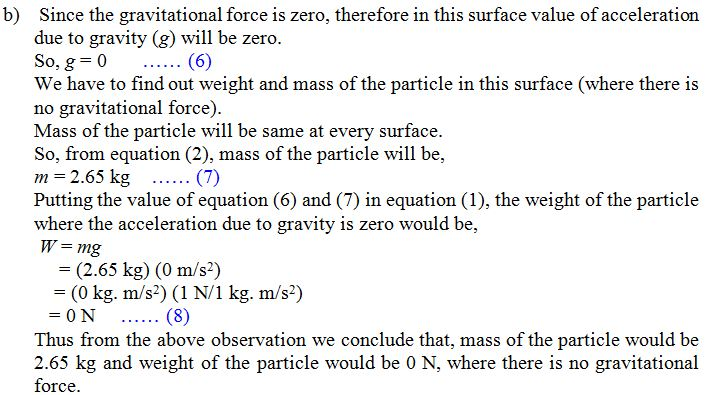×#### Thank you for registering.

One of our academic counsellors will contact you within 1 working day.

Click to Chat

1800-1023-196

+91-120-4616500

CART 0

• 0

MY CART (5)

Use Coupon: CART20 and get 20% off on all online Study Material

ITEM
DETAILS
MRP
DISCOUNT
FINAL PRICE
Total Price: Rs.

There are no items in this cart.
Continue Shopping
```
A certain particle has a weight of 26.0 N at a point where the acceleration due to gravity is 9.8 m/s. (a) What are the weight and mass of the particle at a point where the acceleration due to gravity is 4.60 m/s 2 ? (b) What are the weight and mass of the particle if it is moved to a point in space where the gravitational force is zero?
A certain particle has a weight of 26.0 N at a point where the acceleration due to gravity is 9.8 m/s. (a) What are the weight and mass of the particle at a point where the acceleration due to gravity is 4.60 m/s2? (b) What are the weight and mass of the particle if it is moved   to a point in space where the gravitational force is zero?

```
5 years ago396 Points
```Rounding off to three significant figures, weight of the particle would be, 12.2 N.Thus from the above observation we conclude that, mass of the particle would be 2.65 kg and weight of the particle would be, 12.2 N.```
5 years ago
Think You Can Provide A Better Answer ?

## Other Related Questions on Mechanics

View all Questions »### Course Features

• 101 Video Lectures
• Revision Notes
• Previous Year Papers
• Mind Map
• Study Planner
• NCERT Solutions
• Discussion Forum
• Test paper with Video Solution### Course Features

• 110 Video Lectures
• Revision Notes
• Test paper with Video Solution
• Mind Map
• Study Planner
• NCERT Solutions
• Discussion Forum
• Previous Year Exam Questions